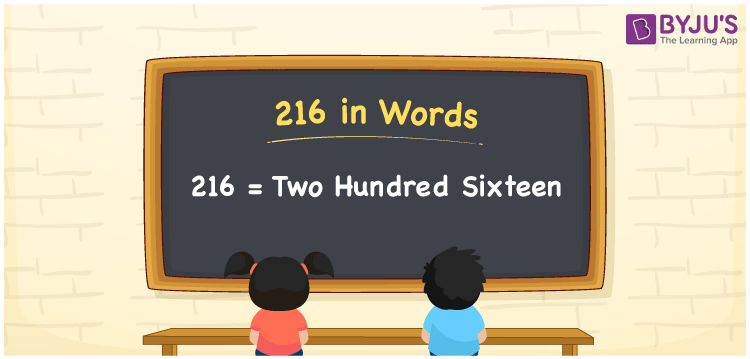# 216 in Words

216 in words is written as Two Hundred Sixteen. The name of number 216 in English is “Two Hundred Sixteen”. The word Two Hundred Sixteen is used in many real-life situations. For example, there are Two Hundred Sixteen students in a classroom. Thus, it is a counting number. Also, to represent the currency equal to 216, we can write it in words as Rupees Two Hundred Sixteen or Two Hundred Sixteen rupees. Therefore, it is necessary to learn the numbers in words, for the ease of understanding and expressing them.

 216 in words Two Hundred Sixteen Two Hundred Sixteen in Numbers 216

## 216 in English Words## How to Write 216 in Words?

If we know the place value of digits of 216, then we can easily express it in words. The place value is basically the position of a digit in a number. 216 is a three-digit number, therefore, we can specify the position of each digit of 216 in a place value chart. In the Indian numbering system, the order of place value of digits from right to left is given by:

 Hundreds Tens Ones 2 1 6

We can write it in expanded form as:

2 × Hundred + 1 × Ten + 6 × One

= 2 × 100 + 1 × 10 + 6 × 1

= 200 + 10 + 6

= 216

= Two Hundred Sixteen

Therefore, 216 in words is written as Two Hundred Sixteen.

216 is a natural number that precedes 217 and succeeds 215.

216 in words – Two Hundred Sixteen

Is 216 an odd number? – No

Is 216 an even number? – Yes

Is 216 a perfect square number? – No

Is 216 a perfect cube number? – No

Is 216 a prime number? – No

Is 216 a composite number? – Yes

## Frequently Asked Questions on 216 in Words

Q1

### How to write 216 in words?

216 in English is written as “Two Hundred Sixteen”.
Q2

### Is 216 an odd number or even number?

216 is an even number because it is wholly divisible by 2.
Q3

### Write Two Hundred Sixteen in numbers.

Two Hundred Sixteen in numbers is 216.